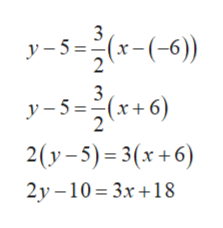GRAPHS AND FUNCTIONSWriting an equation in slope-intercept form given the slope and a...3A line passes through the point (-6, 5) and has a slope of2Write an equation in slope-intercept form for this line.?X

Question

See attachedhelp_outlineImage TranscriptioncloseGRAPHS AND FUNCTIONS Writing an equation in slope-intercept form given the slope and a... 3 A line passes through the point (-6, 5) and has a slope of 2 Write an equation in slope-intercept form for this line. ? X fullscreen
Step 1

The point-slope equation is,

Step 2

Substitute the values x1 = –6, y1 = 5 and m = 3/2 in the distance fo...help_outlineImage Transcriptionclose3 y5=(x(-6) 3 y5=(x+6 2 2(y-5) 3(x+6) 2y -10 3x18 fullscreen

Want to see the full answer?

See Solution

Want to see this answer and more?

Our solutions are written by experts, many with advanced degrees, and available 24/7

See Solution
Tagged in

Other